# Feature selection

## Definition

Feature selection is the process of selecting the set of features of a given input datum that we will use to predict the corresponding output value. For instance, a set of features that we may use to predict the price of a house may be the number of floors, floor area, zip code, size of front porch, and number of windows.

## Distinction between elementary feature selection and derived feature selection

Elementary features are features that cannot be deduced from other, simpler features already available. Derived features are features that can be deduced from other features that have already been included. The choice of derived features can be thought of as more a model class selection rather than a feature selection problem, because derived features can be incorporated into the functional form rather than thought of as features. Therefore, this page concentrates on the selection of elementary features.

## Features and the ontology of examples

In supervised learning, the input data are called examples. One may wonder if examples can be thought of as a vector of its elementary features, in an extensional sense. For instance, a model for house price might use the length$\ell$ and breadth$b$ of each house. In this case, can we just call the vector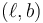$(\ell, b)$ a house?

It is true that in this model, the vector$(\ell, b)$ captures all of the relevant information about the house. However, note the following difficulties with trying to mentally equate the house with the vector:

• Non-uniqueness of representation: We can represent the house in different ways. We could have chosen different elementary features, such as length$\ell$ and area$A$, so that each house is represented as a vector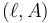$(\ell, A)$, where we can still retrieve$b = A/\ell$ as a derived feature. In other words, each vector is a representation of the house.
• Incompleteness of representation: The house may have other relevant features that are not included in the model. For instance, perhaps features such as the height of the house, or its geographic location, could affect the price. Even though our current model ignore those features, it is still important to remember their existence, and to not equate the house with the particular partial representation we have chosen for a given modeling exercise.

## Considerations in feature selection

### Predictive power

The goal of feature selection is to choose features that can help with predicting the output. Therefore, we should try to select features that can help with predicting the output. Note that the prediction of the output is done by the features in combination, rather than by any one feature individually, but a quick-and-dirty analysis of the sensitivity of the output to individual features can be a good rule-of-thumb for determining whether to include those features.

One approach might be to use a very simple predictive model (such as univariate linear regression or univariate logistic regression) to see how the output varies with a single feature, then use the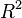$R^2$ or a goodness-of-fit measure to determine whether the feature is worth including. However, this approach might miss features that tend to be predictive only when combined with other features.

### Cost of data-gathering for training examples

A supervised learning algorithm that determines the parameters for the functional form determining the output in terms of the features needs to have access to the values of the features for all the training data (semi-supervised algorithms can make do with the values for a subset of the training data). The cost of this data-gathering can be a constraint in deciding whether to include the feature or not.

In addition to the cost, the precision and accuracy of the feature value as measured also matter.

### Cost of data-gathering for new instances where the value needs to be predicted

In order for a feature to be helpful for a predictive model, its value should be easy to compute for new instances where we are trying to make predictions. Therefore, features whose values are known only after a time lag (relative to when the prediction needs to be made) are not useful for predictive models, even if we have access to past data and can build a retrospectively predictive model using them. One example of features whose values are known only after a time lag is the various economic indicators (unemployment, GPD, etc.) used when predicting economic facts. Here, even if it is possible to predict an economic fact perfectly given the economic indicators, it would still not be possible to have real-time predictions.

## The predictive power may be constrained by the choice of features, regardless of the power of models or learning algorithms

Once the set of features is chosen, that puts an upper bound on just how predictive the model can be. Suppose, for instance, that the output that we are trying to predict is the sum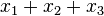$x_1 + x_2 + x_3$ of independent features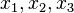$x_1,x_2,x_3$ where$x_3$ is normally distributed with mean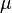$\mu$ and standard deviation$\sigma$. If we choose only$x_1$ and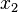$x_2$ as our features, then the most we can say about the output is that it is normally distributed with mean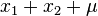$x_1 + x_2 + \mu$ and standard deviation$\sigma$. We simply cannot get more precise.

## Selecting too many features could be problematic

The following are the problems with selecting too many features:

• Collecting information on the values of the features for all the training data, as well as for the new inputs on which we are trying to make predictions, becomes harder.
• The learning algorithm used to optimize the parameter values becomes more computationally intensive.
• A functional form that uses too many features may suffer from overfitting.